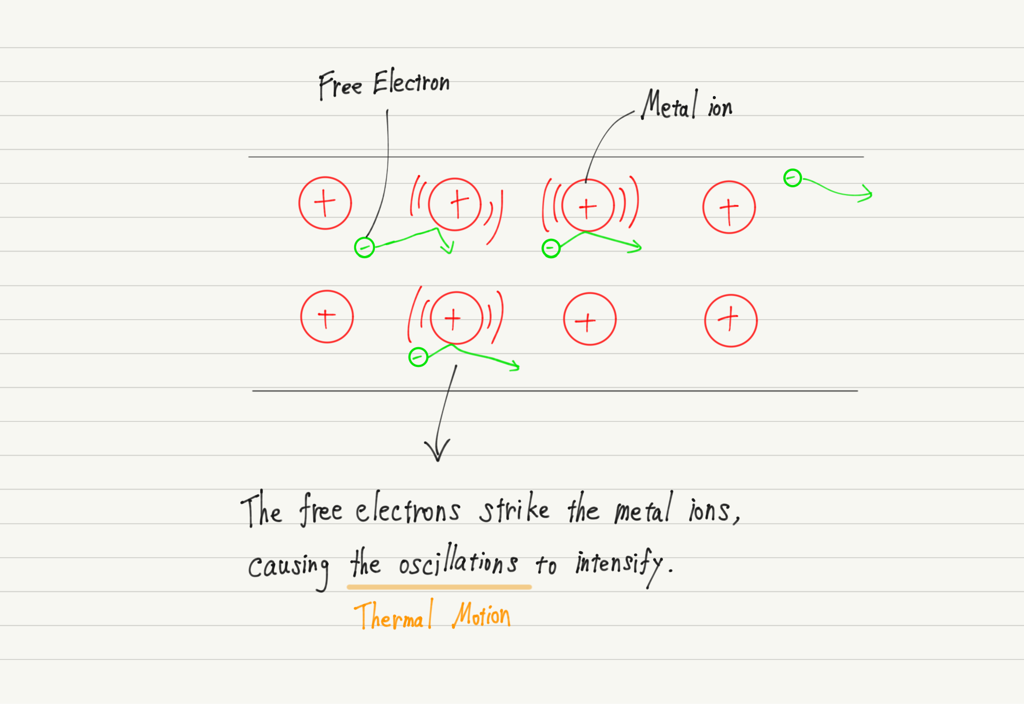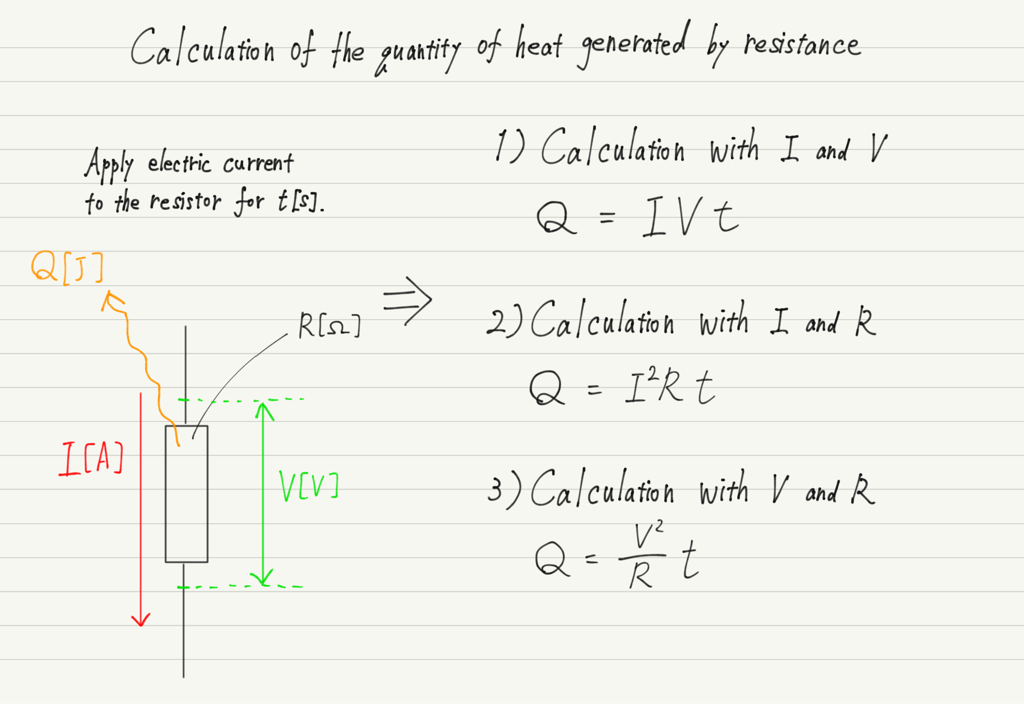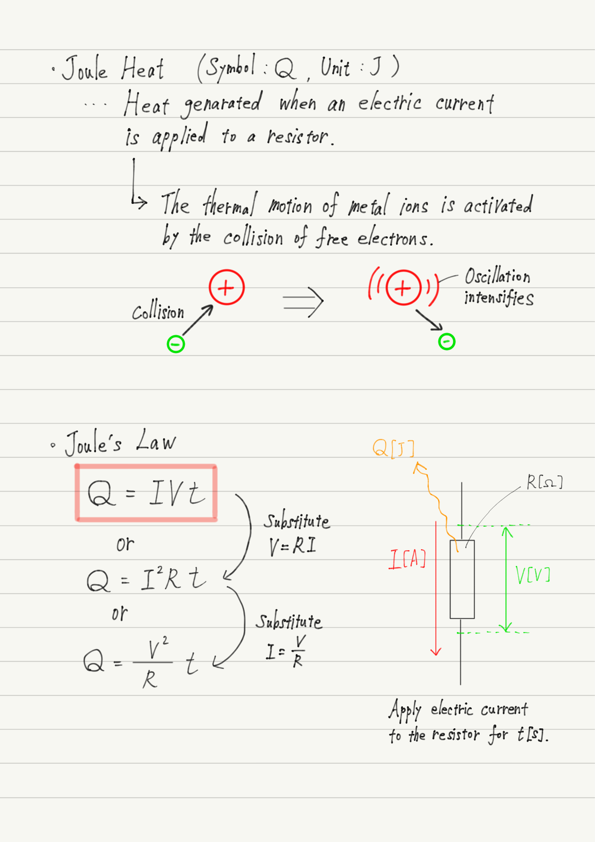# Joule’s Law

(This article was translated from Japanese to English using DeepL.)

A light bulb is a device that converts electrical energy into light energy, but as it glows, it also generates heat. In this lecture, we will consider this phenomenon.

## Why resistance generates heat

Resistance was the image of a “hard path to get through”.

https://www.yukimura-physics.com/entry/elemag-f04

The reason for the difficulty is that the cations of the metal prevent the free electrons from progressing.

The free electrons proceed by colliding with metal ions. This collision imparts free electron kinetic energy to the metal ion, causing it to vibrate more violently than before the collision.Remember what you learned in thermodynamics.
Vibration of atoms composing a solid = Thermal motion

For more information, see the following article.
https://www.yukimura-physics.com/entry/therm-f01
The kinetic energy of the free electrons passing through the resistance is the energy of thermal motion of the metal ions. This is how the resistance generates heat.

## Calculation of calorific value by Joule’s law

So how much heat is actually generated when a current flows through a resistor?

This varies depending on the magnitude of the current, the value of the resistance, and the voltage applied, and this was investigated by a man named Joule. His name is also a unit of energy.

According to the joule, the amount of heat generated in resistance can be calculated by the formula shown below.The equation of these relations is called “Joule’s law” and the heat generated is called Joule heat.

Joule’s law states that the amount of heat can be calculated if two of I, V, and R are known.

However, since Ohm’s law holds for I, V, and R, it is not necessary to memorize all three equations.
Remember the equation Q = IVt, and the others can be derived using Ohm’s law.

## Summary of this lecture## Next Time

The next lecture will be the last one on electromagnetism in Basic Physics. The final theme is power consumption.

https://www.yukimura-physics.com/entry/elemag-f10

error: Content is protected !!